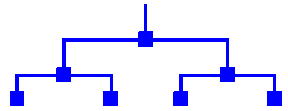# binary tree

(redirected from Full binary tree)

## binary tree

[′bīn·ə·rē ′trē]
(mathematics)
A rooted tree in which each vertex has a maximum of two successors.
McGraw-Hill Dictionary of Scientific & Technical Terms, 6E, Copyright © 2003 by The McGraw-Hill Companies, Inc.

## binary tree

(btree) A tree in which each node has at most two successors or child nodes. In Haskell this could be represented as

data BTree a = NilTree | Node a (BTree a) (BTree a)

## binary tree

A data structure in which each node contains one parent and no more than two children. See quad tree and splay tree.

Binary TreeCopyright © 1981-2019 by The Computer Language Company Inc. All Rights reserved. THIS DEFINITION IS FOR PERSONAL USE ONLY. All other reproduction is strictly prohibited without permission from the publisher.
References in periodicals archive ?
Let T be a full binary tree with depth d (1 [less than or equal to] d [less than or equal to] n), where the leaf stands for user.
Algorithm B initials a full binary tree T of depth d (1 [less than or equal to] d [less than or equal to] n), and all the node in T is numbered from 1 to ([2.sup.d] - 1).
From the full binary tree T, user path is denoted as path(uid) = [mathematical expression not reproducible]}.
Binary tree with n nodes, the full binary tree or a complete binary tree with minimum path length, the length of the binary tree with the right path:
The full binary tree [T.sub.h] of height h can be defined as the rooted plane tree such that:
A binary tree is a subtree of a full binary tree with the same root.
We consider finite full binary trees in which every node has zero or two children.

Site: Follow: Share:
Open / Close